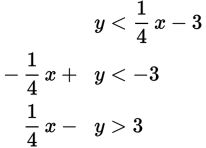# SAT Math Multiple Choice Question 355: Answer and Explanation

### Test Information

Question: 355

10.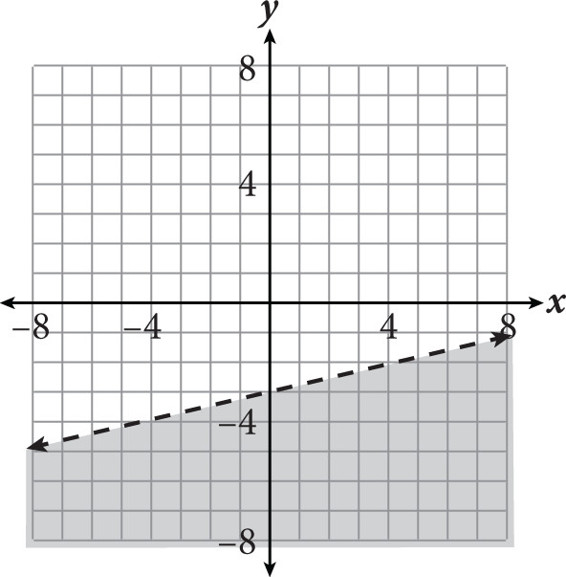The solution to which inequality is represented in the graph above?

• A.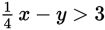• B.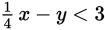• C.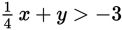• D.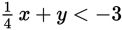Explanation:

A

Difficulty: Medium

Category: Heart of Algebra / Inequalities

Strategic Advice: Don't answer this question too quickly. The shading is below the line, but that does not necessarily mean that the symbol in the equation will be the less than symbol (<).

Getting to the Answer: Start by writing the equation of the dashed line shown in the graph in slope-intercept form. Then use the shading to determine the correct inequality symbol. The slope of the line shown in the graph is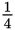and the y-intercept is -3, so the equation of the dashed line is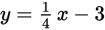. The graph is shaded below the boundary line, so use the < symbol. When written in slope-intercept form, the inequality is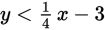. The inequalities in the answer choices are given in standard form (Ax + By = C), so rewrite your answer in this form. Don't forget to reverse the inequality symbol if you multiply or divide by a negative number.